# NEET Mock Test - 3

## 180 Questions MCQ Test NEET Mock Test Series | NEET Mock Test - 3

Description
Attempt NEET Mock Test - 3 | 180 questions in 180 minutes | Mock test for NEET preparation | Free important questions MCQ to study NEET Mock Test Series for NEET Exam | Download free PDF with solutions
QUESTION: 1

### In the base of an npn transistor, ______ are the minority carriers.

Solution:
• The electrons in the base are called minority carriers because the base is doped p-type, which makes holes the majority carrier in the base.
• In an npn transistor, the majority charge carriers in base is holes and minority charge carriers are electrons.

NPN Transistor: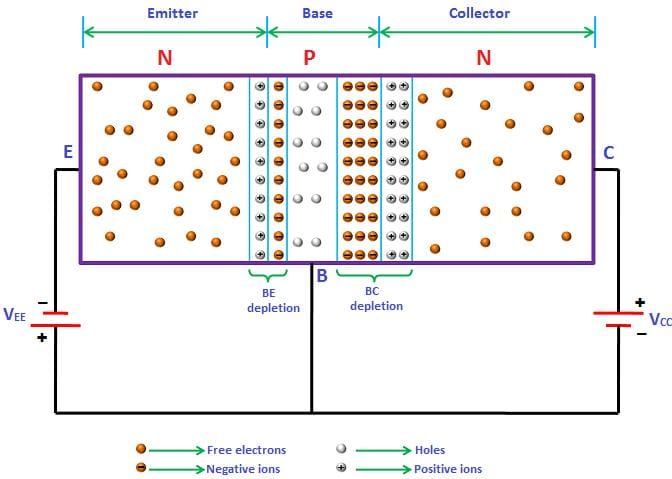QUESTION: 2

### The volume of a cube in m3 is equal to the surface area of the cube in m2. The volume of the cube is:

Solution:

Considering each edge length be 'a'.
Then, volume of cube =
Also, surface area of the same cube = 6a²
According to the question, both of them are equal.
i.e., ►  a³ = 6a²
This brings us to the conclusion, a = 6m.
Thus, volume of cube is 216 m³.

QUESTION: 3

### A ball is released from the top of a tower of height h metres. It takes T seconds to reach the ground. What is the position of the ball in T/3 seconds?

Solution:

► The acceleration of the ball will be g.
Initial velocity will be 0.
In T sec body travels h metres.

► By applying equations of motion we get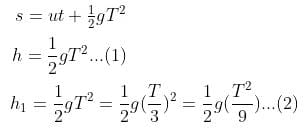► from (1) and (2) we get h1 ​= h/9 distance from point of release.

Distance from the ground is: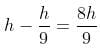QUESTION: 4

In a uniform circular motion (horizontal) of a ball tied with a string, velocity at any time is at an angle θ with acceleration, then θ is:

Solution:

In a uniform circular motion, acceleration is always towards center and velocity is along the tangent. So, θ=90.

QUESTION: 5

Two masses A and B each of mass M are fixed together by a massless spring. A force acts on the mass B as shown in fig. At the instant shown, the mass A has acceleration ‘a’. What is the acceleration of mass B?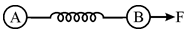Solution:

Body A has acceleration a.
Let acceleration of B be b.
► Mb = F – T
where T = Ma
► Mb = F – Ma
► b = {(F – Ma) / M}
b = [(F / M) – a]

QUESTION: 6

The surfaces are frictionless, the ratio of T1 and T2 is: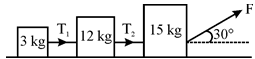Solution:

According to Newton's second Law,
► T1 = m1 a
► T2 = (m1 + m2)a
► (T1 / T2) = {m1 / (m1 + m2)}
= {3 / (3 + 12)} = (1 / 5)

Hence, T1 : T2 = 1:5

QUESTION: 7

Two blocks of masses M1 and M2 are connected with a string passing over a pulley as shown in fig.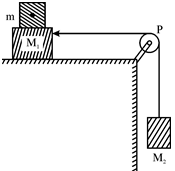The block M1 lies on a horizontal surface. The coefficient of friction between the block M1 and the horizontal surface is μ. The system accelerates. What additional mass m should be placed on the block M1 so that the system does not accelerate?

Solution:

For the equilibrium of block of mass M:
Frictional force, f = tension in the string, T
where T = f = µ(m + M1)g   ...(i)
For the equilibrium of block of mass M:
► T = M2g     ...(ii)
From Eqs. (i) and (ii), we get μ(m + M1)g = M2g
► m = M2/μ - M1

QUESTION: 8

Six marbles are lined up in a straight groove made on a horizontal frictionless surface as shown below. Two similar marbles in contact, with a common velocity v collide with a row of 6 marbles from left. Which of the following is observed?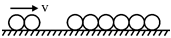Solution:
• According to the law of conservation of linear momentum when two bodies of same mass collide they exchange velocities.
• ∴ Only two marbles from the extreme right will roll with a speed 'V' and the other balls will remain at rest.
QUESTION: 9

The power of water pump is 2 kW. If g = 10 m / s2, the amount of water it can raise in 1 minute to a height of 10 m is:

Solution:

Given:
Power = 2kw = 2000w
Height H = 10m
Time taken t = 60 seconds
Now,
► Power = Energy/time = mgh / t
2000 = m*10*10 / 60
We get m = 1200 kg
Therefore, the pump lifts 1200 kg or 1200 litres of water to a height of 10m every minute.

QUESTION: 10

If kinetic energy is doubled, find fractional change in momentum?

Solution:

Kinetic energy is:
K = (1/2) mv2
We want to write it in form of momentum. We know that momentum, P = mv
Multiply and divide by m,
► K = (1/2) m2 v2 / m
► K = (1/2) (mv)2 / m
► K = (P2) / 2m
Thus Kinetic Energy is proportional to the square of momentum.
Also, P = √(2mK)
So, momentum is proportional to the square root of kinetic energy.
Thus, if kinetic energy is doubled, then momentum will become √2 times the original momentum.

QUESTION: 11

When a particle of mass m rotating in a plane circular path of radius r has an angular momentum L. Calculate centripetal force acting on it?

Solution:

Centripetal force = mv2/r
Angular momentum = mvr = L
Thus centripital force,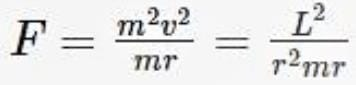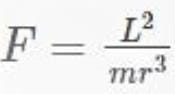QUESTION: 12

A metre scale is standing vertically on earth’s surface on one of its ends. It now falls on the earth without slipping. Find the velocity with which the free end of the scale strikes the earth g = 9.8 m/s2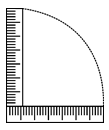Solution:

According to the law of conservation of energy, as no non-conservative external or internal force acts upon the system, energy is conserved, thus we have,
mgh = ½ I w2
where I is moment of inertia and w is angular speed.
I = mL/ 3 and w = v / L
Thus we get,
► mgL = mv2 / 3
Thus we get v = √3gL
Thus we get v = 5.4m/s

QUESTION: 13

The height vertically above the earth’s surface at which the acceleration due to gravity becomes 1% of its value at the surface is (R is the radius of the earth)______.

Solution: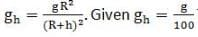∴  we have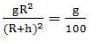► R + h = 10 R
► h = 9R, which is option B.

QUESTION: 14

A particle of mass 10 g is kept on the surface of a uniform sphere of mass 100 kg and radius 10 cm. Find the work to be done against the gravitational force between them to take the particle far away from the sphere [Given G = 6.67 × 10–11Nm2/kg2) ].

Solution: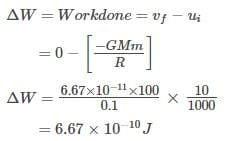QUESTION: 15

For a wire of length l, the maximum change in length under stress condition is 2 mm. What is the change in length under the same conditions when length of wire is halved?

Solution:

From Hooke's law, if the strain is small then stress is proportional to strain, i.e.,
stress = Y×strain
where Y is a proportionality constant called Young's modulus.
Young's modulus is given by: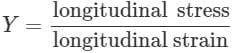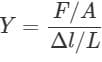where F is force, A is area.
Δl is change in length and L is original length. First case,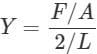... (i)
Second case,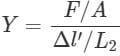... (ii)
Equating Eqs. (i) and (ii), we get: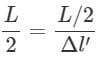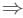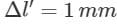QUESTION: 16

A ball of mass m and radius r is released in viscous liquid. The value of its terminal velocity is proportional to:

Solution:

From the equation of terminal velocity we have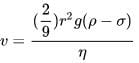Here we see that: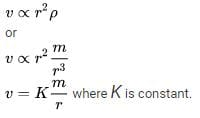QUESTION: 17

The pressure inside two soap bubbles is 1.01 and 1.02 atmosphere respectively. The ratio of their respective volumes is:

Solution:

Excess pressure(P) in first drop = 1.01 - 1 = 0.01 atm
Excess pressure in second drop = 1.02 - 1 = 0.02 atm

Pressure × Radius = Surface Tension
Here the pressure is Gauge Pressure, i.e the difference between absolute pressure and atmospheric pressure
Hence ratio of gauge pressures is 1:2
Accordingly ratio of radii is 2:1
Hence ratio of volumes is 8:1
P is inversely proportional to r(radius). So ratio of their volumes will be:
► r2/ r1= (0.02)/ (0.01)3 = 8 : 1

QUESTION: 18

A beaker is completely filled with water at 4oC. It will overflow if ______.

Solution:
• Density of water is maximum at 4°C and density will decrease if we cool it above 4°C or heat above 4°C.
• So for mass to remain constant, the volume should increase and when volume increases, water overflows.
QUESTION: 19

Two wires A and B are of the same material. Their lengths are in the ratio 1: 2 and the diameter are in the ratio 2:1. If they are pulled by the same force, then increase in length will be in the ratio:

Solution:

We know,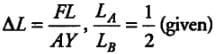[∵ The wires A and B are pulled by the same force and they are made up of same material hence, FA = FB = F, YA = YB = Y]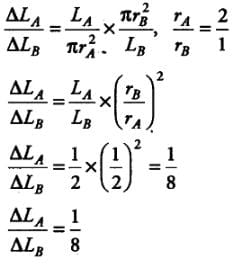QUESTION: 20

Find the change in internal energy of the system when a system absorbs 2 kilo-calorie of heat and at the same time does 500 joules of work.

Solution:

► ΔQ = ΔU + ΔW
Since, ΔQ = 0 (in Adiabatic Process)
► ΔU + ΔW = 0
► ΔU = ΔQ - W
► ΔQ = 2 K cal = 2 x 4.2 x 1000 J = 8400 J
► W = 500 J
∴ ΔU = (8400 - 500) J = 7900 J

QUESTION: 21

The pressure inside a tyre is 4 times that of the atmosphere. If the tyre bursts suddenly at a temperature of 300 K, what will be the new temperature?

Solution: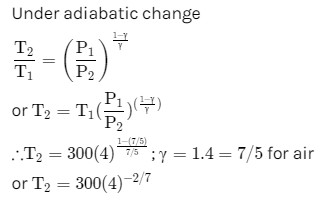QUESTION: 22

Air is filled at 60°C in a vessel of an open mouth. The vessel is heated to a temperature T so that 1/4th part of the air escapes. Assuming the volume of the vessel remaining constant, the value of T is:

Solution:If pressure and volume of gas remains constant then MT = constantQUESTION: 23

Molar specific heat at constant volume, Cv for a monoatomic gas is:

Solution:

According to first law of thermodynamics,
ΔQ = ΔU + PΔV
If ΔQ is absorbed at constant volume, ΔV = 0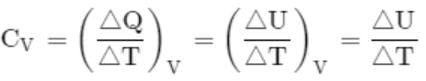for an ideal monoatomic gas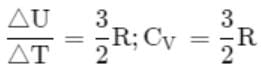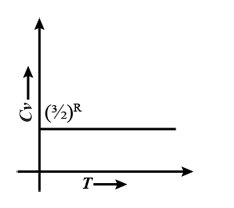QUESTION: 24

To make the frequency double of a spring oscillator, we have to:

Solution: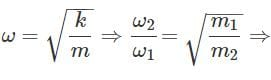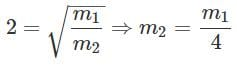QUESTION: 25

The displacement-time graph of a particle executing S.H.M. is as shown in Fig.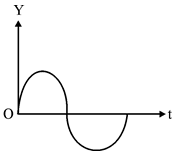Solution:

Acceleration =−w2y.
So, F = - mw2y is sinusoidal function.
So, F will be also a sinusoidal function with phase difference p.

QUESTION: 26

For the stationary wave,
y = 4 sin (πx / 15) cos (96 πt)
the distance between a node and the next antinode is:

Solution:

Given , y = 4sin(πx / 15) cos 96 πt
For nodes, displacement is zero.
∴ sin(πx/15) ​= 0
⇒ πx / 15​ = 0, π, 2π...
⇒x = 0, 15, 30...
For antinodes, displacement is maximum.
∴ sin(πx/15) ​= 1
⇒ (πx/15)​ = 2π​, 23π​...
⇒ x = 15/2​, 45/2​
Hence the difference between node and antinode = 15/2 ​− 0 ​= 7.5 m

QUESTION: 27

A train is moving at a constant speed along a circular track. The engine of the train emits a sound of frequency n. The frequency heard by the guard at the rear end of the train is:

Solution:

As we know according to the Doppler effect the speed of the listener and source are the same so both of them heard the same frequency.

QUESTION: 28

Eight dipoles of charges of magnitude 'e' are placed inside a cube. The total electric flux coming out of the cube will be:

Solution: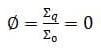i.e., net charge on dipole is zero.

QUESTION: 29

Two condensers, one of capacity C and the other of capacity C/2, are connected to a V volt battery, as shown.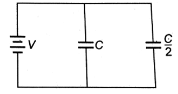The work done in fully charging both the condensers is:

Solution:

The two condensers in the circuit are in parallel order, hence:
► C' = C + (C/2) = 3C/2

The work done in charging the equivalent capacitor is stored in the form of potential energy.
► W = U = C'V/ 2
= 3CV/ 4

QUESTION: 30

The resistance between the terminal points A and B of the given infinitely long circuit will be: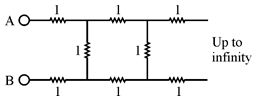Solution:

The equivalent circuit may be shown as:Where R may be treated as the total resistance.QUESTION: 31

8 identical cells, each of emf E and internal resistance r, are connected in series. If polarity of two cells is reversed, then the total internal resistance in the circuit will be:

Solution:
• Resistance is the inherent property of a body and is independent of its alignment, so even if the polarity is reversed, the resistance will be same.
• Here, emf of each cell = E
Internal resistance of each cell = r
• Total internal resistance of 8 cells connected in series is: rseries = 8r
• If the polarity of two cells are reversed, then the total internal resistance of the cells is: r′series = 8r
i.e., there is no effect on the total internal resistance of the cells.
QUESTION: 32

In a metre bridge, the balancing length from the left end (standard resistance of one ohm is in the right gap) is found to be 20 cm. The value of the unknown resistance is:

Solution:

Metre bridge works on the principle of Wheatstone bridge.QUESTION: 33

Consider three long, straight, parallel wires shown in fig. The force experienced by 25 cm length of wire C is: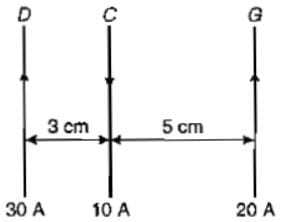Solution:

Repulsion by wire D ...... (towards right)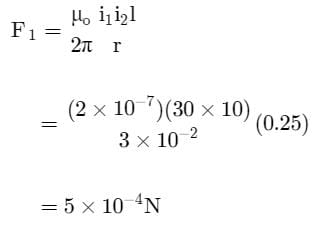Repulsion by wire G ....... (towards left)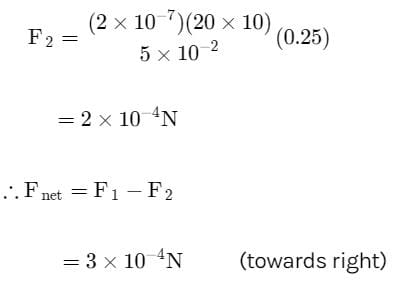QUESTION: 34

The magnetic field at a distance d from a short bar magnet in longitudinal and transverse position are in the ratio:

Solution:

► B1(longitudinal) = 2M/d3
► B2(transverse) = M/d3
► B/ B2 = 2 : 1

QUESTION: 35

If the self-inductance of 500 turn coil is 125 mH, then the self-inductance of similar coil of 800 turns is:

Solution:

Self-inductance L =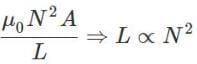Or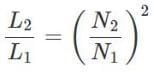Or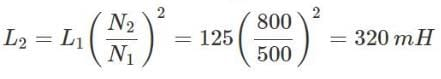QUESTION: 36

A transformer of efficiency 90% draws an input power of 4 kW. An electrical appliance connected across the secondary draws a current of 6 A. The impedance of the device is:

Solution:

We know that Efficiency, η = Po/Pi
Where Po is output power and Pi is input power.
Therefore, the output power is given by
Po = η Pi = 0.9 × 4000 W = 3600 W.
Now as the power factor is 90% thus we get impedance almost equal to resistance and as
► P = I2R
► R =  P/I2
= 3600 / 36
= 100 Ω

QUESTION: 37

In an electromagnetic wave, the amplitude of electric field is 1V/m. The frequency of wave is 5 × 1014 Hz. The wave is propagation along Z-axis. The average energy density of electric field in joule/m3, will be:

Solution:

Average energy density of electric field is given by: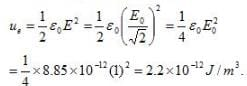QUESTION: 38

A point source of electromagnetic radiation has an average power output of 800 W. The maximum value of electric field at a distance 3.5 m from the source will be:

Solution:

Intensity of EM wave is given by, I = P/4πR2
► vac⋅c = 1/2∈0E02 × c
► E0 = (P/2πR20c)1/2
= (800 / (2 * 3.14 * (3.5)* 8.85 * 10-12 * 3 * 108))1/2
= 62.6 V/m

QUESTION: 39

A glass slab (μ = 1.5) of thickness 6 cm is placed over a paper. What is the shift in the letters?

Solution: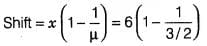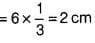QUESTION: 40

A spherical mirror forms an erect image three times the linear size of the object. If the distance between the object and the image is 80 cm, the focal length of the mirror is:

Solution:

Image size = 3 × object size
∴ m = (v/u) = 3
Given: u + v = 80 cm
► u + 3u = 80 cm
► u = 20 cm
► v = 60 cm as image is erect, image must be virtual.
► u = -20 cm
► v = 60 cm
Using (1/v) + (1/u) = (1/f)
► (1/f) = (1 / 60) – (1 / 20)
► (1/f) = (– 2 / 60) = (– 1 / 30)
► f = – 30 cm
∴ Concave mirror with f = 30 cm.

QUESTION: 41

Radiations of two photons energies, twice and five times the work function of metal are incident successively on the metal surface. The ratio of the maximum velocity of photoelectrons emitted in the two cases will be:

Solution: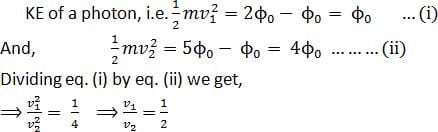QUESTION: 42

An electron and a proton are possessing same amount of kinetic energy. The de-Broglie wavelength is greater for:

Solution:

De Broglie wavelength = h/mv
Since wavelength is inversely proportional to mass and electron having less mass will have a greater wavelength.

QUESTION: 43

In the nuclear reaction,
92U239 93Np239 + X
X stands for:

Solution:
• It is a case of Beta decay. In the above reaction, an electron and antineutrino are emitted.
• Here mass number remains the same but the atomic number is increased by 1 which can be balanced by the -1 charge of electron so that number remains the same in LHS and RHS.
QUESTION: 44

Fusion reactions take place at high temperature because ______.

Solution:

When the coulomb repulsion between the nuclei is overcome then nuclear fusion reaction takes place. This is possible when temperature is too high.

QUESTION: 45

A disc is rolling (without slipping) on a horizontal surface. C is its centre and Q and P are two points equidistant from C. Let vP, vQ and vC be the magnitudes of velocities of points P, Q and C respectively, then: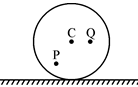Solution:

The rolling (without slipping) about O is shown in figure. From figure OQ > OC > OPWe know that , v = rω

vQ> vC>vP

QUESTION: 46

2-­Methylbutane on reacting with bromine in the presence of sunlight gives mainly:

Solution:
• The reactivity order of abstraction of H atoms towards bromination of alkane is 3°H (tertiary) > 2°H (secondary) > 1°H (primary).
• Bromine will be substituted to the Hydrogen where carbon has least number of hydrogen atoms. In this case, methyl group is attached at second position, so only 1 hydrogen is attached to second carbon atom (tertiary).
QUESTION: 47

Standard Gibbs free energy change for a reaction is zero. The value of the equilibrium constant will be:

Solution:

∆G = - RTlnK
For ∆G to be zero, lnK should be zero, i.e., K = 1.

QUESTION: 48

In an amino acid, the carboxyl group ionises at pKa1= 2.34 and ammonium ion at pKa2 = 9.6. The isoelectric point of the amino acid is at pH:

Solution:

The isoelectric point of the amino acid is at pH = (pKa1 + pKa2) / 2
= (2.34 + 9.6) / 2 = 5.97

QUESTION: 49

Apparatus to measure the Rate of Respiration and Respiratory Quotient is:

Solution:

A respirometer is a device used to measure the rate of respiration of a living organism by measuring its rate of exchange of oxygen and/or carbon dioxide.

QUESTION: 50

Consider the following compounds: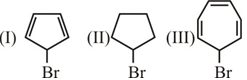What is the correct sequence of the compound given above in decreasing order of their SN1 reacting?

Solution:
• Carbocation formed by I will be anti-aromatic and highly unstable. So it won't participate in SN1 reaction.
• Carbocation in II will be more stable than I and it will participate in the reaction with moderate reactivity.
• Carbocation in III will be aromatic and so participate in the reaction with the highest reactivity.
• ​So the order is III > II > I.
QUESTION: 51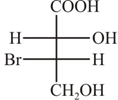What is the absolute configuration of chiral centre 2 and 3 of the compound given above?

Solution:
QUESTION: 52

Which of the following is most acidic?

Solution:

The compound can easily lose H+ ion because it gains the most stable structure that is aromatic structure because it has 6πe in resonance and as we know that the compound which loses H+ ion easy is more acidic in nature.

QUESTION: 53

Which of the following compound on strong heating evolves ammonia gas?

Solution:

Ammonia is obtained by heating ammonium sulphate.
(NH4​)2​SO​→ NH​+ NH4​HSO4

QUESTION: 54

Name of the compound given below is: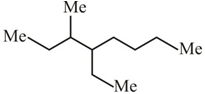Solution:
QUESTION: 55

In the reaction: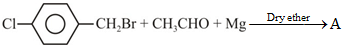, product A is:

Solution:
QUESTION: 56

When NaCl is doped with 1.0 x 10-3 mol % of SrCl2, the concentration of cation vacancies will be?

Solution:
• NaCl is doped with 10-3 mole % of SrCl2. This means, 100 moles of NaCl are doped with 10-3 moles of SrCl2.
• Therefore, 1mole of NaCl is doped with 10-3 /100 = 10-5 moles of SrCl2 or 10-5 moles of Sr2+.
• Now, One Sr2+ ion creates one cation vacancy. Therefore, no. of cation vacancies created by 10-5 moles Sr2+ = 10-5 moles / mole of NaCl
= 10-5 x 6.022 x 1023 / mole of NaCl
= 6.022 x 1018/ mole of NaCl
QUESTION: 57

Which one of the following amines will give carbylamine reaction?

Solution:

Ethylamine reacts with chloroform (CHCl3) & Alcoholic KOH (KOH in ethanol), then ethyl isocyanide is formed which is a bad-smelling compound.
C2H5NH2 + CHCl3 + 3KOH(alc.) ⇒ C2H5NC (ethyl isocyanide) + 3KCl + 3H2O

QUESTION: 58

The concentration of Ag+ in a standard solution of AgCl was found to be 10-5 mol L-1. At the same temperature the concentration of Ag+ in a 0.01 M sodium chloride solution saturated with AgCl will be:

Solution:
QUESTION: 59

Consider the following statements associated with the reaction: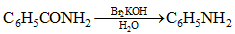Solution:
• In this reaction, the analyte is heated with alcoholic potassium hydroxide and chloroform.
• If a primary amine is present, the isocyanide (carbylamine) is formed, as indicated by a foul odour.
QUESTION: 60

Given the following bond energy values:
C - C = 348 kJ / mol
C - H = 413 kJ / mol
C - O = 357 kJ / mol
The enthalpy of formation (in kJ / mol-1) of gaseous dimethyl ether from a gaseous atom is:

Solution:

Dimethyl ether has 6 C-H bonds and 2 C-O bonds. (zero C-C bonds)
Enthalpy of formation = 6*413 + 2*357 = 3192 KJ/mol-1

QUESTION: 61

The second ionisation potential of C, N, O and F are such that:

Solution:

The correct order of second ionisation potential of carbon, nitrogen, oxygen, fluorine is:
O > F > N > C
► Nitrogen: [He] 2s2 2p3
► Oxygen: [He] 2s2 2p4
► Fluorine: [He] 2s2 2p5
► Carbon: [He] 2s2 2p
After removing one electron:​

• Nitrogen: [He] 2s2 2p2 - After the removal of one more electron, it will have only one electron in its outermost p subshell.
• Oxygen: [He] 2s2 2p3  - After removal of one more electron, it will have two electrons in p subshell which makes it very unstable. So have very high IP.
• Fluorine: [He] 2s2 2p- After removal of one more electron, it will occupy a stable half-filled configuration.
• Carbon: [He] 2s2 2p1 - After the removal of one more electron, it will occupy the ideal gas configuration. Hence it will easily give away second electron having a very low second IP.
QUESTION: 62

In the reaction,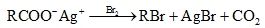"In the statement, this reaction is known as X reaction and proceeds via a Y intermediate", X and Y stand respectively for:

Solution:
• The Hunsdiecker reaction is a name reaction in organic chemistry whereby silver salts of carboxylic acids react with a halogen to produce an organic halide.
• The intermediate formed is organic free radical.
QUESTION: 63

In the reaction,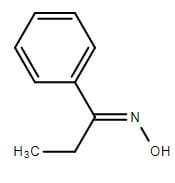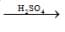Solution:
QUESTION: 64

Among the following the least thermally stable is:

Solution:

Li2CO3 decomposes on heating.
Li2CO3 → Li2O + CO2

QUESTION: 65

The unit cell of a binary alloy composed of A and B metals has a CCP structure with A atoms occupying the corners and B atoms occupying centres of each face of the cube. If during the crystallization of their alloy, in the unit cell two A atom are missed, the overall composition per unit cell is:

Solution:
• Corner atoms in a unit cell have contributions  = 1 / 8 each
• Face centred atoms have a contribution of = 1 / 2 each
• Total No. of atoms of A in CCP = (1 / 8) x (8 - 2) = (1 / 8) x 6 = 3 / 4 atoms
• Total no. of atoms of B atoms = (1 / 2) x 6 = 3 atoms
• Therefore molecular formula is A3/4B3 or AB4
QUESTION: 66

The rate constant for a first-order reactant is 6.909 min-1. Therefore, the time required in minutes for the participation of 75% of the initial reactant is:

Solution:

For first order reaction,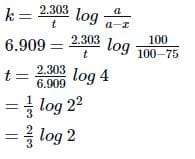QUESTION: 67

At 300 K two pure liquids A and B have vapour pressures respectively 150 mm Hg and 100 mm Hg. In an equimolar liquid mixture of A and B, the mole fraction of B in the vapour mixture at this temperature is:

Solution:

Let the moles of A = x
∴ The moles of B = x (∵ mixture is equimolar)
Mole fraction of A =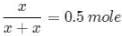= Mole fraction of B
Thus,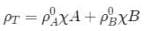ρT = 150 x 0.5 + 100 x 0.5 = 125
∴ Mole fraction of B in the vapour mixture =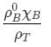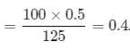Hence, option D is correct.

QUESTION: 68

In a reversible reaction, the enthalpy change and the activation energy in the forward direction are respectively -x kJ mol-1 and y kJ mol-1.Therefore the energy of activation in the backward direction in kJ mol-1 is :

Solution:
QUESTION: 69

The most powerful oxidising agent of the following is:

Solution:

Fluorine hydration energy is very high due to its small size.

Also, dissociation enthalpy of fluorine is less as compared to chlorine due to large electron-electron repulsion in fluorine.

Because of these factors, fluorine has high positive electrode potential than chlorine.

Hence, among halogens, F2 is strongest oxidising agent.

QUESTION: 70

Which one of following is non-reducing?

Solution:

Order of volatility  H2Te >  H2Se > H2S > H2O

​Water has the highest boiling point and thus is least volatile.

QUESTION: 71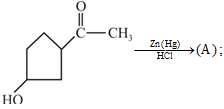Solution:
QUESTION: 72

An alkene on ozonolysis yield only ethanal. There is an isomer of this, which on ozonolysis yields:

Solution: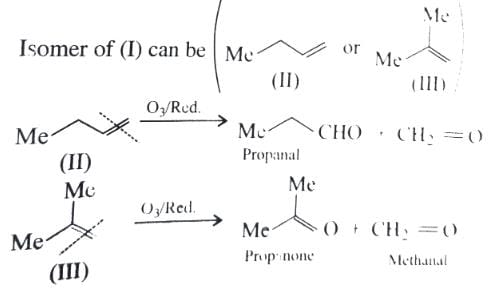QUESTION: 73

A mixture of Benzaldehyde and Formaldehyde on heating with aqueous NaOH solution gives:

Solution:

Crossed Cannizzaro's reaction where formaldehyde is oxidized and benzaldehyde is reduced. This occurs because aliphatic are oxidized much more readily compared to aromatic aldehydes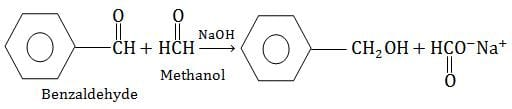QUESTION: 74

A new carbon-carbon bond formation is possible in:

Solution:

In Reimer-Tiemann reaction. Here new C-C bond formed between carbon of benzene ring and -CHO group.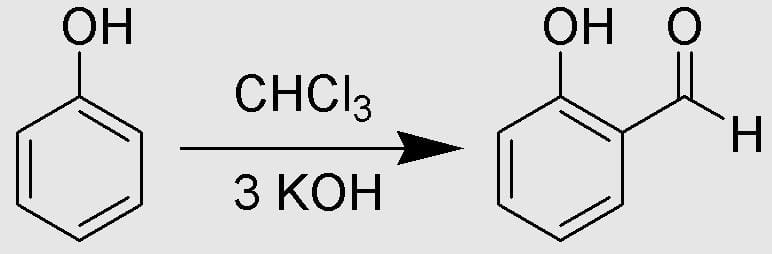QUESTION: 75

Among the given structure which can exhibit tautomerism ?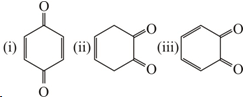Solution:
QUESTION: 76

What volume of methane (NTP) is formed from 8.2 gm of sodium acetate by fusion with soda lime :

Solution:
QUESTION: 77

In the following sequence of reactions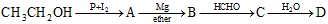the compound D is :

Solution:
QUESTION: 78

The end product 'R' of the following series of reaction is :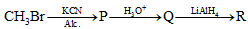Solution:
QUESTION: 79

When n–chloro benzaldehyde is treated with 50% KOH solution, the product (s) obtained is (are)?

Solution: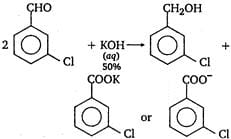QUESTION: 80

The correct order of decreasing acid strength of trichloroacetic acid (a), trifluoroacetic acid(B), acetic acid(C) and formic acid (D) is :

Solution:

If an electron withdrawing group (-I showing group) is present, it makes the removal of proton more easy by stabilising the remaining carboxylate ion and thus, makes the acid more acidic. The order of acidity of given compounds is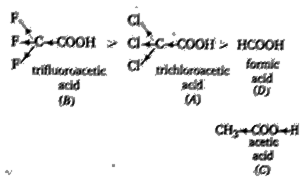QUESTION: 81

The no. of moles of KMnO4 that will be needed to react with one mole of sulphate ion in acidic solution is :

Solution:

2MnO4 - +H++5SO32-->5SO4 2-+2Mn 2+

So, one mole of sulphate ion will need 2/5 moles of KMnO4.

QUESTION: 82

Select the rate law that corresponds to the date shown far the following reaction :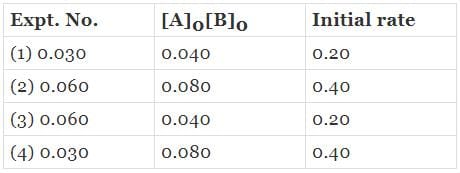Solution:
QUESTION: 83

In view of the signs of (ΔrG0) for the following reactions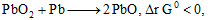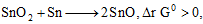which oxidation states are more characteristic for lead and tin ?

Solution:

The tendency to form +2 ionic state increase on moving down the group due to the inert pair effect.

Most characteristic oxidation state for lead and tin are +2,+4 respectively.

QUESTION: 84

The heat of neutralisation of strong base and strong acid is 57.0 kJ. The heat released when 0.5 mole of HNO3 , solution is added to 0.20 mole of NaOH solution is :

Solution:

Heat of neutralization (ΔH)-57 kJ/mol, moles of HNO= 0.5 mole and moles of NaOH= 0.2 mole. When HNO3 solution is added to NaOH solution, then 0.2 mole of HNO3 solution will combine with 0.2 mole of NaOH solution.

Heat released = ΔH x 0.2= 57 x 0.2= 11.4 kJ

QUESTION: 85

The value of λ0eq for NH4Cl, NaOH and NaCl are respectively 149.74, 248.1 and 126.4 ohm-1 cm2 equi-1. The value of λ0 eq for NH4OH is :

Solution:

Λoeq​ or Λ(NH4​OH)​=Λ∞​eq(NH4​Cl) + Λ∞​eq(NaOH)−Λ∞​eq(NaCl)

= (149.74+248.1−126.4)

= 271.44 ohm−1cm2eq−1

QUESTION: 86

Match the List-1 with List-2 and select the correct answer from the given data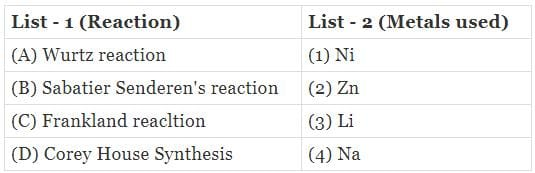Solution:

Wurtz reaction sodium/dry ether,

Sabatier senderen's=nickel/H2,

Frankland=zinc,

Corey house = lithium/copper.

QUESTION: 87

The decreasing order of strength of the bases,

OH-,  NH2,  H-C≡C- and  CH3-CH2is:

Solution:

Strong base has its weak conjugate acid.
Strength of conjugate acid:
H2O > CH≡CH > NH​> CH3​CH3
So strength of base follow an reverse order as:
CH3-CH2> NH2- > CH≡C  >OH-

QUESTION: 88

The reaction of propene with HOCl proceeds via the addition of :

Solution:

Alkenes undergo electrophilic addition reactions.HOCl on self ionisation produces Cl+ which attacks first.
HOCl + HOCL → H2O + OCl- + Cl+
∴The option is (b) Cl+ in the first step.

QUESTION: 89

Methyl acetate on treating with excess of C5H5MgBr Produces :

Solution:
QUESTION: 90

Inflorescence of Ficus is:

Solution:
• Hypanthodium: In this type of inflorescence the receptacle forms a hollow cavity with an apical opening guarded by scales. Here the flowers are borne on the inner wall of the cavity.
• The flowers are unisexual; the female flowers develop at the base of the cavity and the male flowers towards the apical pore.
• The examples are found in genus Ficus of Moraceae family, Example: Ficus carica, F. glomerata, F. benghal- ensis, F. religiosa, etc.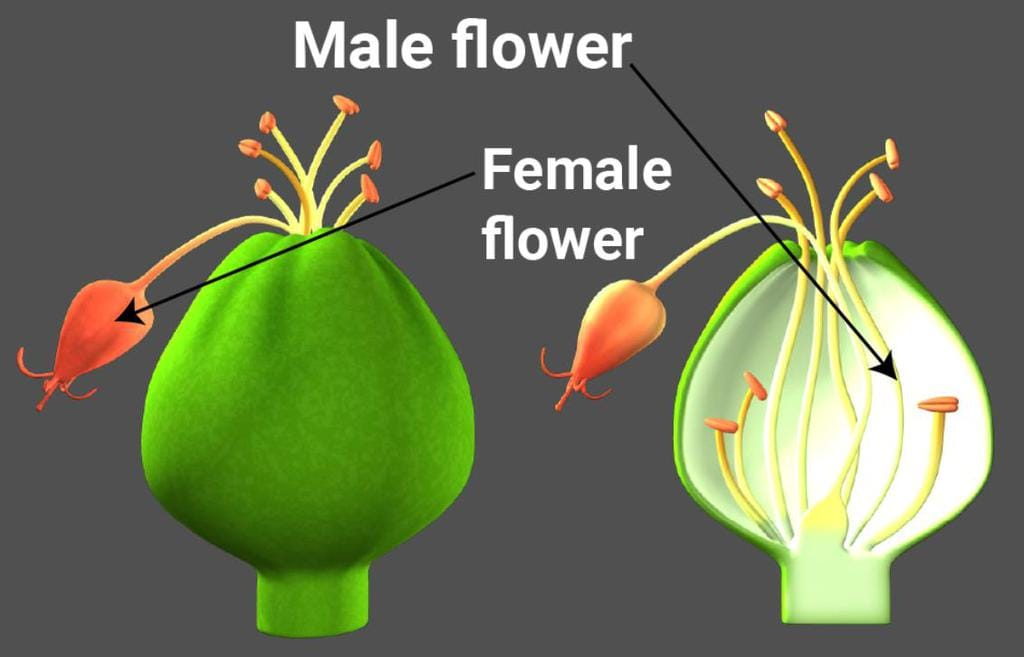QUESTION: 91

Verticillaster is the modified form of:

Solution:
• A reduced raceme or cyme that grows in the axil of a bract is called a fascicle.
• verticillaster is a fascicle with the structure of a dichasium; it is common among the Lamiaceae.
• Many verticillasters with reduced bracts can form a spicate (spike-like) inflorescence that is commonly called a spike.
QUESTION: 92

Which part is edible in litchi?

Solution:

The edible part of litchi fruit is 'aril'. The litchi fruits consists of peel, aril and seed.

QUESTION: 93

Syngenesious anthers and epipetalous stamens are found in:

Solution:

In Asteraceae or Compositae, stamens are 5, epipetalous and alternate. The stamens are syngenesious that is only anthers are fused into a tube around the style and filaments are free. Anthers are dithecous, basifixed and dehiscing longitudinally.
Syngenesious anthers and epipetalous stamens: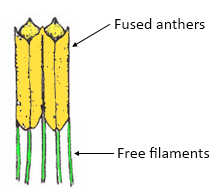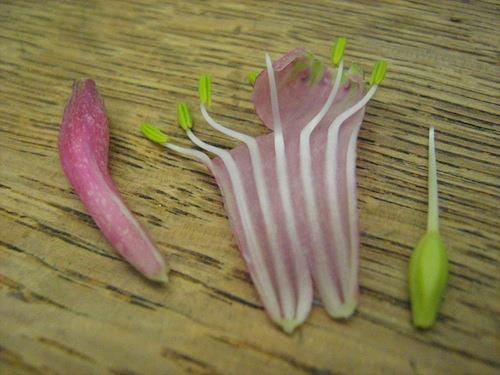QUESTION: 94

Embryo is:

Solution:
• Embryo consists of a scutellum and a short embryo axis (tigellum). The scutellum is a shield-shaped cotyledonattached to a node of embryo axis.
• The surface of scutellum facing endosperm is called epithelial layer. Hence the correct option is C
QUESTION: 95

When pollination takes place by Ants is:

Solution:

Entamophily --- insects

Ornithophily --- birds

Cheriptophily --- bats

Myrmicophily --- ants.

QUESTION: 96

Conjoint, collateral, open vascular bundle is found in:

Solution:

The vascular tissue is present in the form of strands known as the vascular bundles.

Characteristics of vascular bundle of a dicot stem:

• Conjoint- when xylem and phloem are present on the same radius.
• Collateral- when phloem is usually present on the outerside of xylem . But in few families, for example Cucurbitaceae, Solanaceae, Apocynaceae phloem also differentiates on the inner side of xylem and this is called as internal phloem.

Dicot stem: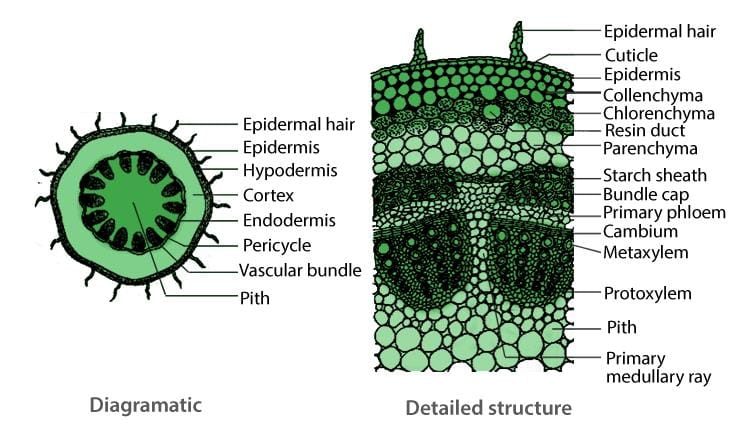QUESTION: 97

Bulliform apparatus is found in:

Solution:

In dicot leaves, bulliform cells are absent. They contain three types of cells in epidermis and these are- epidermal cells, guard cells, subsidiary cells. When these cells also lose water, the leaf may fold but not as much as in case of monocot leaves containing bulliform cells.

QUESTION: 98

In Dracana which type of vascular bundle is found:

Solution:

A vascular bundle in which xylem encircles the central strand of phloem is known as amphivasal bundle also called leptocentric bundle.
Examples: Dracaena, Yucca.

QUESTION: 99

End product of glycolysis is ______.

Solution:
• Glycolysis is a metabolic process in which one glucose molecule is converted into 2 private molecule under aerobic condition.
• The end product of glycolysis are 4 ATP molecule, out of which 2 ATP molecule are utilized, 2 pyruvic acid molecule and 2 NADH molecule.
• Thus, the correct answer is 'pyruvic acid'.
QUESTION: 100

Net gain of ATP in Glycolysis with electron transport chain :

Solution:

Two molecules of ATP and two molecules of NADH, for every molecule of glucose is broken down.The net gain of ATP is 8 (including NADH).

QUESTION: 101

Chemosynthetic bacteria obtain energy from:

Solution:
• In Inorganic Chemicals Chemosynthetic bacteria were the first organism on earth synthesise their own food by obtaining energy from chemicals like H2S,NO2, etc.
• Photosynthetic bacteria have taken their origin from Chemosynthetic bacteria. Sun, infrared rays and organic substances are not used as the sources of energy for Chemosynthetic bacteria.
QUESTION: 102

Energy required for ATP synthesis in PS1 comes from?

Solution:
• The proton gradient is formed due to accumulation of protons on the lumen side of the thylakoid.
• Also, the transport of electrons through the photosystem moves protons from the stroma to the lumen side, decreasing the proton concentration in the stroma and increasing it on the lumen side.
• This results in the generation of a proton gradient across the thylakoid membrane. The ATPase enzyme breaks down this proton gradient and uses its energy for the synthesis of ATP molecules.
QUESTION: 103

Mitochondria are called powerhouses of the cell which of the following observations support this statement?

Solution:
• Mitochondria are double membrane-bound structures and are the site of ATP production which is the energy currency of the cell.
• The rest of the statements, though are correct but, does not verify and support the fact that mitochondria are the powerhouses of the cell.

Mitochondria: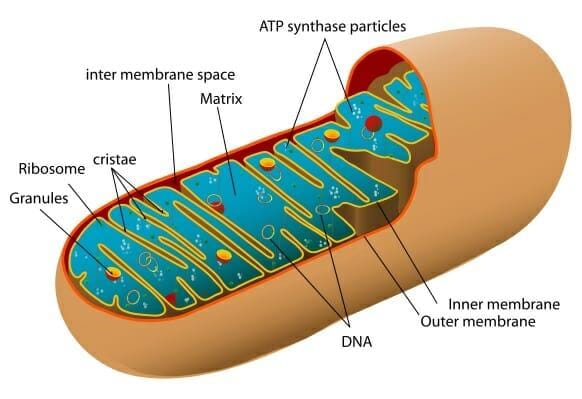QUESTION: 104

The ultimate electron acceptor of respiration in anaerobic organism is:

Solution:
• In aerobic organisms undergoing respiration, electrons are shuttled to an electron transport chain, and the final electron acceptor is oxygen.
• Molecular oxygen is a highly oxidizing agent and, therefore, is an excellent electron acceptor.
QUESTION: 105

Splitting of water is associated with?

Solution:
• Light reactions occur inside the thylakoids, especially those of grana region. It involves two types of reactions photolysis of water and production of assimilatory power.
• The phenomenon of breaking up of water into hydrogen and oxygen in the illuminated chloroplasts is called photolysis or photocatalytic splitting of water.
• Water splitting complex is associated with the PSII, which itself is physically located on the inner surface of thylakoid membrane.
QUESTION: 106

When a plant undergoes senescence the nutrients may be ?

Solution:

When a plant undergoes senescence, mineral ions are frequently mobilized from older parts to apical and lateral meristems, young leaves, developing fruits and seeds by diffusion and by active uptake by cells in the growing regions. This is known as translocation of nutrients.

So, the correct answer is option B.

QUESTION: 107

Match the following and choose the correct option: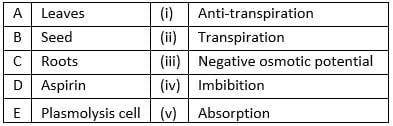Solution:
QUESTION: 108

Reaction carried out by N2 fixing microbes include.

A. 2NH3 + 3O→ 2NO2- + 2H+ + 2H2O......(i)

B. 2NO2 + O→ 2NO3-..........(ii)

Which of the following statements about these equations is not true?

Solution:

Phototrophic bacteria are a group of bacteria, whose energy for growth is derived from sunlight and their source of carbon comes from carbon dioxide or organic carbon.

So, the correct option is 'Bacteria carrying out these steps are usually photoautotrophs'.

QUESTION: 109

Which one of the following will not directly affect transpiration?

Solution:

The chlorophyll content of leaves will not directly affect transpiration, while temperature, light and wind speed directly affect the transpiration.

QUESTION: 110

Plasticity in plant growth means that?

Solution:

Different kinds of structures develop in plants in different phases of growth or in response to environment. This ability is called plasticity.

QUESTION: 111

Persistent calyx is found in:

Solution:

The members of the family Solanaceae have persistent calyx. It includes plants like potato, brinjal etc.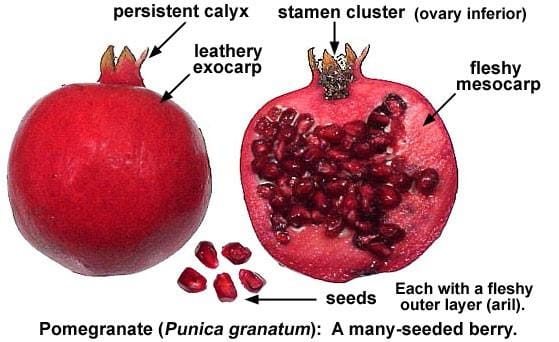QUESTION: 112

Organisms living in salty areas are called as?

Solution:

Halophiles: Bacteria living in extremely salty areas.
• Thermoacidophiles: Bacteria living in hot springs/deep seawater.
Example: Thermococcus
• Methanogens: Bacteria living in marshy areas and produce methane gas.
Heliophytes: Sun-loving plants.

QUESTION: 113

All eukaryotic unicellular organisms belong to:

Solution:
• Protista is a group of all unicellular eukaryotic plants and animals. The organisms included in this group are either photo- autographs, heterotrophs or parasites.
• On the other hand, Monera includes prokaryotic like bacteria, unicellular organism Fungi are eukaryotic but are mostly multicellular (exception yeast is unicellular).
QUESTION: 114

The five kingdom classification was proposed by:

Solution:
• The five kingdom classification was proposed by R.H. Whittaker in 1969. The five kingdoms were formed on the basis of characteristics such as cell structure, mode of nutrition, source of nutrition and body organisation.
• It includes Kingdom Monera, Kingdom Protista, Kingdom Fungi, Kingdom Plantae, and Kingdom Animalia.
QUESTION: 115

Botanical gardens and Zoological parks have?

Solution:

Botanical gardens and zoological parks are specialised places where living plants are grown and living animals are kept (where they can reproduce too) respectively. Both, endemic (local), as well as exotic (foreign to that place) species, are kept in these places.

QUESTION: 116

The embryosac of an Angiosperm is made up of?

Solution:

Embryo sac of an angiosperm contains 8 nuclei but 7 cells -3 micropylar, 3 chalazal and one central cell. The central cell is the largest cell and contains two polar nuclei.

Mature embryo sac of angiosperm: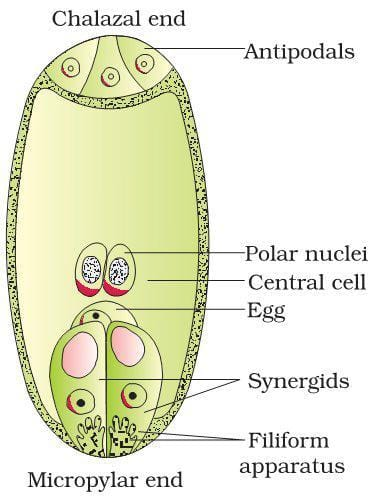QUESTION: 117

Fusion of two gametes which are dissimilar in size is termed as:

Solution:
• Fusion of two gametes which are of dissimilar size is called anisogamous.
• Fusion of flagellate gametes of similar size is called isogamous. Oogamous is the fusion between one large, non - motile female gamete with a smaller, motile male gamete.
• Agamous does not involve the fusion of male and female gametes in reproduction.
QUESTION: 118

Hold fast, stipe and frond constitute the plant body in case of:

Solution:

Phaeophyceae in the members of class-Phaeophyceae, the plant body is usually attached to the substratum by a hold fast and has a stalk called stipe and a leaf like photosynthetic organ called frond.

QUESTION: 119

Protonema is ______ .

Solution:
• The predominant stage of the life cycle of a moss is the gametophyte which consists of two stages.
• The first stage is the protonema stage (juvenile stage) and the second stage is the leafy stage.
• Moss protonema resembles multicellular green algae in structure. Moss plant develops from protonema.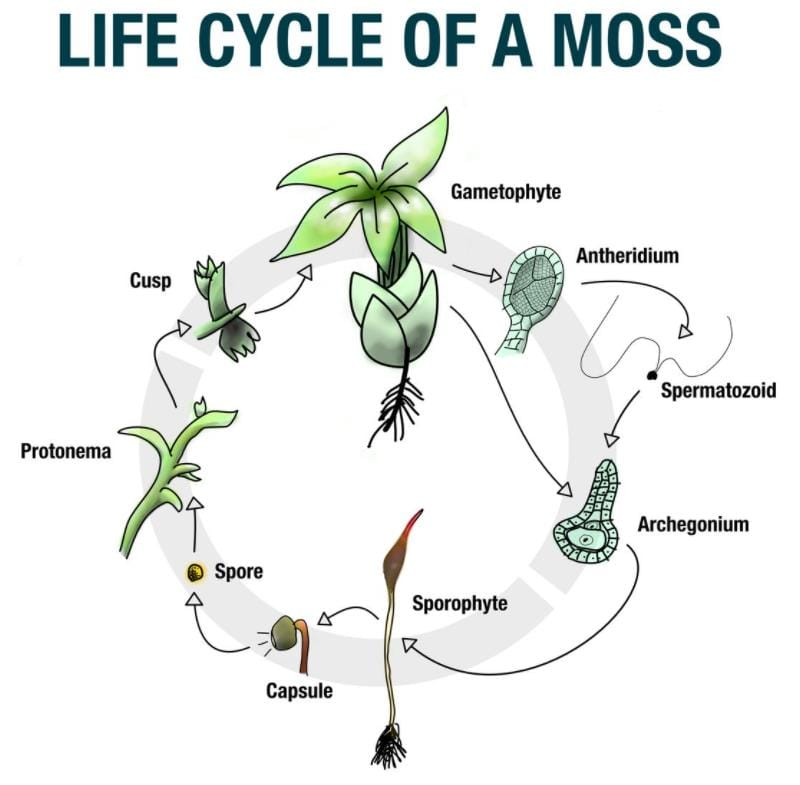QUESTION: 120

The male gametes of rice plant have 12 chromosomes in their nucleus. The chromosome number in the female gamete, zygote and the cells of the seedling will be, respectively:

Solution:
• In the female gamete, the chromosome number will be same as that of the male gamete (12). A zygote is a fertilized egg/seed which means gametes from the parents have been combined (diploid) and thus, the chromosome number will be 24 (2n).
• A seedling is a young plan; sporophyte developing out of a plant embryo from a seed. So, the chromosome number in the cells of the seedlings will be 24 (2n), which will further give rise to new diploid individual.
QUESTION: 121

The statement given below describe certain features that are observed in the pistil of flowers:

(a)  Pistil may have many carpels

(b)  Each carpel may have more than one ovule

(c)  Each carpel has only one ovule

(d)  Pistil have only one carpel

Choose the statements that are true from the option below:

Solution:
• A pistil, (one a many carpels) has three parts ,i.e., stigma, style and ovary.
• Ovary, the swollen part of the pistil contains an angiospermic, ovoid and whitish structure called ovule.
• Inside ovary, it is attached to a parenchymatous cushion called the placenta, either singly or in cluster.

Flower Anatomy:QUESTION: 122

Which of the following pairs of asexual reproductive structures is found in animals?

Solution:

Gemmule, in this type of asexual reproduction, internal buds, called gemmules are involved. Gemmules are asexually reproduced mass of cells, that is capable of developing into a new organism.
Example: Sponges.

QUESTION: 123

Choose the correct statement from the following:

Solution:
• The pollination that occurs in opened flowers is called chasmogamy. It is the most common type of pollination in all types of flowers.
• Chasmogamy is of two types i.e., self-pollination (autogamy) and cross-pollination. Cross-pollination is of two types i.e., geitonogamy and xenogamy. So, we can say that chasmogamous flowers exhibit both autogamy (self-pollination)and allogamy (cross-pollination).
• While in Cleistogamous flower the anthers and stigma lie close to each other with in the closed flowers. When anthers dehisces in the flower buds, pollen grains come in contact with the stigma for effective pollination.
• Thus, these flowers are invariably autogamous as there is no chance of cross-pollen landing on the stigma.
QUESTION: 124

In an embryo sac, the cells that degenerate after fertilization are:

Solution:

In an embryo sac, the cells that degenerate after fertilization are Synergids and antipodals.

QUESTION: 125

Mendel’s law of independent assortment holds good for genes situated on the:

Solution:
• According to the Mendel's law of independent Assortment, the pairs of factors segregate independently of each other when germ cells are formed.
• Homologous chromosomes synapse during meiosis and then separate to segregate independently into different cells which establishes the quantitative basis for segregation and independent assortment of hereditary factors.
QUESTION: 126

The inheritance pattern of a gene over generation among humans is studied by pedigree analysis. Character studied in the pedigree analysis is equivalent to:

Solution:
• Mendelian inheritance in humans is difficult to study. Current understanding of mendelian inheritance in humans is gained by analysis of family pedigrees or the results of matings that have already occured.
• By analyzing a pedigree, we may be able to predict how the trait is inherited. It is a visual tool for documenting the biological relationship in families and to determine the mode of inheritance (dominant, recessive etc.,) of genetic diseases.
• Whereas quantitative trait, polygenic trait and maternal traits are not studied by pedigree analysis.
• Continuous traits are often measured and given a quantitative value, they are often referred as quantitative traits, e.g., crop yield, weight, gain in animals, IQ, etc.
• Polygenic traits are another exception to mendels rule, which occurs when a trait is controlled by more than one gene. This means that each dominant allele adds to the expression of the next dominant allele. Maternal traits are the traits inherited and expressed from the maternal parent to the subsequent offsprings.
QUESTION: 127

It is said that Mendel proposed that the factor controlling any character is discrete and independent. This position was based on the:

Solution:
• Mendel proposed that the factor Controlling any character is discrete and independent.
• His proposition was based on the observations that the offspring of a cross made between the plants having two contrasting characters shows only one character without any blending.
QUESTION: 128

The net electric charge on DNA and histones is:

Solution:
• The net electric charge on DNA and histones is negative and positive.
• DNA molecule is negatively charged due to the presence of phosphate ions in the nucleotides.
• The histones are positively charged due to presence of lysine and arginine amino acid residues.
QUESTION: 129

At which phase of meiosis, the two cells, each with separated sister chromatids move towards opposite poles?

Solution:

The two sister chromatids of each chromosome are captured by microtubules from opposite spindle poles. In metaphase II, the chromosomes line up individually along the metaphase plate. In anaphase II, the sister chromatids separate and are pulled towards opposite poles of the cell.

Meiosis II :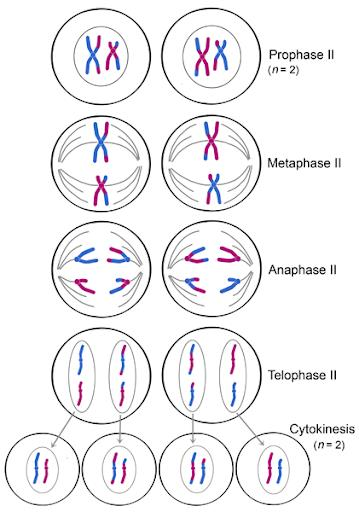QUESTION: 130

The free living fungus Trichoderma can be used for ______ .

Solution:

Trichoderma species are free living and very common in the root ecosystems. So they are effective biocontrol agents of several plant pathogens.

QUESTION: 131

Potatoes are vegetatively propagated from:

Solution:
• Potato is an underground modified stem. Potatoes mostly reproduce in vegetative manner.
• Potatoes have eyes like structure called nodes or buds. Shoots that come out from the buds are called sprouts which grow and become stems.
• They come out after a long period of dormancy. Roots also come out from the buds. The buds become new plants.
• The part of the stem grows under the soil is called underground stem. This underground stem starts swelling and transform into tuber or new potato. When the tubers are fully grown the mother plant dies.
• Nodes of tuber again give rise to buds and new plants as well.
QUESTION: 132

In 2005, for each of the 14 million people present in a country 0.028 were born and 0.008 died during the year, using exponential equation, the number of people present in 2015 is predicted as:

Solution:

In 2005, for each of the 14 million people present in a country, 0.028 were born and 0.008 died during the year.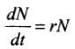r = 0.028 - 0.008 = 0.02
dt = 2015 - 2005 = 10 years
N = N2005 = 14 million
dN = change in population in 10 years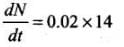dN = 0.02 x 14 x 10
dN = 2.8 milliom
dN = N2015 - N2005
N2015 = dN + N2005
= 2.8 + 14
= 16.8 ≈ 17 million

QUESTION: 133

Productivity is the rate of production of biomass expressed in terms of:
i. (kcalm-3 )yr-1
ii. g-2 y-1 r

iii. g-1yr-1
iv. (kcal m-2) yr-1

Solution:

The rate of synthesis of energy containing organic matter or biomass by any trophic level per unit area in unit time is described as its productivity. It is measured as weight(e.g., g/m2/yr) or energy (e.g., kcal/m2/yr). Hence, only unit(iv) is correct.

QUESTION: 134

Which of the following causes biomagnifications ?

Solution:

Biomagnifications, also called as bioaccumulation is the accumulation of non-biodegradable toxic material like Hg, DDT, etc, in different trophic levels. In the process of bioaccumulation, the concentration of non- biodegradable pollutants increases at each successive trophic level of the food chain, thus harming the environment/ecosystem at an alarming rate, whereas SO2 (sulphur dioxide) is an air pollutant and acts as precursor of acid rain.

QUESTION: 135

During seed germination its store food is mobilized by _______ .

Solution:
• Gibberellin induces aleurone cells to secrete an enzyme to break stored food in seed.
• Cytokinines promote nutrient mobilisation, which helps in the delay of leaf senescence.
• ABA plays an important role in seed development, maturation and dormancy.
• Ethylene induces fruit ripening, breaks seed dormancy.
QUESTION: 136

The epithelial cells lining the stomach of vertebrates are protected from damage by HCI because ______ .

Solution:
• The stomach and intestine are lining by columnar epithelium.
• The cells of columnar epithelium are tall broad at outer end and narrower at inner end.
• The mucus is secreted by this epithelia and protect it from HCL (The cardiac glands secretes mucus).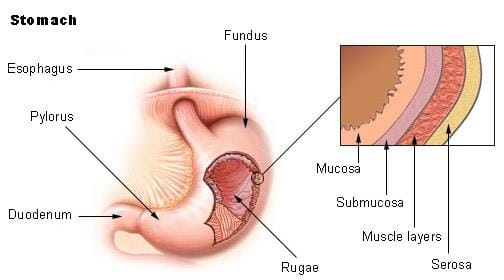QUESTION: 137

Which one of the following is the correct matching of the events occurring during menstrual cycle?

Solution:
• Secretory phase is also called as luteal phase the Luteinising Hormones (LH) is secreted by the anterior lobe of pituitary gland and causes ovulation.
• It stimulates cells of ovarian follicles to develop corpus luteum corpus.
• Luteum secretes large amount of progesterone.
QUESTION: 138

pH of stomach is made 7. Which component of food would be affected?

Solution:
• Acidic pH in stomach is necessary to activate the proteolytic(protein digesting) enzyme pepsin.
• On changing the pH to 7 (basic) ,the pepsin remains in inactivated form and hence protein digestion is affected.
QUESTION: 139

Which one of the following statements is correct?

Solution:
• Malignant tumour first grows slowly. No symptoms are noticed. This stage is called the latent stage. The tumour later grows quickly.
• The cancer cells go beyond adjacent tissue and enter the blood and lymph. Once this happens, they migrate to many other sites in the body, where the cancer cells continue to divide.
• It is metastasis. Only malignant tumours are properly designated as cancer.
QUESTION: 140

Which of the following statements is correct?

Solution:
• Neanderthal and Homo sapiens have a common ancestor, that most people think of as Homo heidelbergensis.
• However, since Neanderthal and Homo sapiens interbred, so another way to think of this is to simply say that we are both just a sub-species of Homo heidelbergensis.
• The ancestor of Homo heidelbergensis is some sort of later stage Homo ergaster.
• So, in another way we can tell that Neanderthal man is the direct ancestor of Homo sapiens.
QUESTION: 141

What will happen if the secretion of parietal cells of gastric glands is blocked with an inhibitor?

Solution:
• Parietal cells are the stomach epithelial cells that secrete gastric acid (HCl) and intrinsic factor. These cells are located in the fundus.
• Gastric HCl activates pepsinogen and converted into the pepsin that breaks the peptide bonds by a process known as proteolysis.
• So, in the absence of HCl secretion, inactive pepsinogen is not converted into the active enzyme pepsin.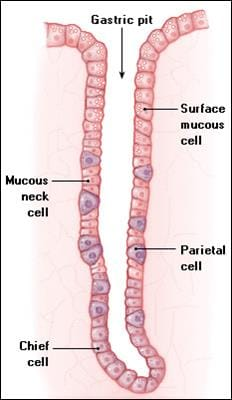QUESTION: 142

Which one of the following statements is true regarding digestion and absorption of food in humans?

Solution:
• Fructose and amino acids are absorbed through intestinal mucosa with the help of carrier ions like Na⁺.
• Carbohydrates are absorbed as monosaccharides (simple sugars such as flucose, fructose, and galactose that cannot be further broken done by hydrolysis) or as disaccharides carbohydrates (such as sucrose, lactrose, maltose, and dextrin that can be hydrolyzed to two monosaccharides).
• These simpler molecules, however, must be obtained by the breaking down of polysaccharides, complex carbohydrates that contain many monosaccharides.
• Chief among these is amylase, a starch that accounts for 20 percent of dietary carbohydrate.
QUESTION: 143

During CO2 transport, HCO3- diffuses from erythrocytes to plasma and in turn upsets the ionic balance momentarily. In order to keep the ionic balance, an equal number of Cl- pass into the erythrocytes from plasma. The process is known as:

Solution:
• About 70% of CO2​ is converted to bicarbonate ions (HCO3​) and transported in plasma. CO2​diffuses into RBCs, combines with water and forms carbonic acid {H2​CO3​). H2​CO3​ being unstable quickly dissociates Into H+ and HCO3​. HCO3​ ions are quite diffusible.
• Therefore, HCO3​ diffuses from RBCs into the plasma. To maintain the ionic balance CI− ions move from the plasma into the RBCs. This exchange is called chloride shift or Hamburger's phenomenon.

So, the correct answer is 'Hamburger phenomenon'.

QUESTION: 144

The signal transduction of steroid hormone across cell is through:

Solution:
• Steroid hormones, being hydrophobic molecules, diffuse freely into all cells and act within the cell. Steroid hormones secreted by adrenal cortex, ovaries, and testes do not utilize the second messenger to exert their influence.
• Steroid hormones enter the cytoplasm of a target cell and bind with specific receptor proteins (mobile).
• Hormone-receptor complex than diffuses into the nucleus and activates specific genes to form a new protein. This protein carries out the specific response for a particular steroid hormone.
QUESTION: 145

Wuchereria bancrofti is transmitted by:

Solution:
• Wuchereria bancrofti is the organism which causes filariasis in humans.
• The parasite completes its life cycle in two hosts. The humans serve as their definitive host.
• The culex mosquitoes are the intermediate host. The organism transmits the disease with the help of culex mosquitoes.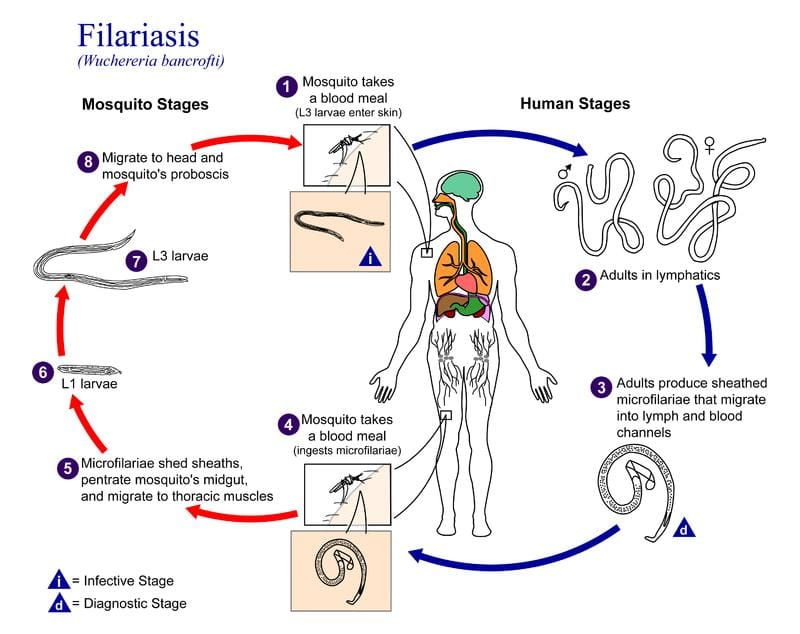QUESTION: 146

Rate of breathing is controlled mainly by:

Solution:
• The rate of breathing is controlled mainly by COlevel in blood. The partial pressure of carbon dioxide controls the respiratory rate.
• The respiratory centre measures the levels of carbon dioxide, oxygen and acidosis in the arterial blood and sends message accordingly.
• If the carbon dioxide levels in the blood increases, the rate and depth of breathing increases.
QUESTION: 147

Podocytes are the cells present in:

Solution:

Podocytes are cells in the Bowman's capsule in the kidneys that wrap around capillaries of the glomerulus. The Bowman's capsule filters the blood, retaining large molecules such as proteins while smaller molecules such as water, salts, and sugars are filtered as the first step in the formation of urine.

QUESTION: 148

Which of the following substances, if introduced into the blood stream, would cause coagulation of blood at the site of its introduction?

Solution:
• Lipoproteinaceous, thromboplastin is released by the injured tissue. This reacts with Ca++  ions present in blood and forms a proteinaceous enzyme called prothrombinase. Later in the presence of Ca++ inactivates heparin (anticoagulant) and catalyses prothrombin (inactive plasma protein) into an active thrombin protein.
• Thrombin acts as an enzyme and catalyses fibrinogen (a soluble plasma protein) into an insoluble fibre like polymer, fibrin.
• These fibres form a dense network upon the wound and trap blood corpuscles (WBCs, RBCs and platelets) and thus form a clot. This clot seals the wound and stop bleeding.
• In blood vessels, thromboplastin do not release due to which blood does not clot. But external thromboplastin to blood will cause blood clotting at the site of its introduction due to formation of prothrombinase.
QUESTION: 149

The heart sound ‘dup’ is produced when:

Solution:
• Lubb sound which is produced at the time of ventricular systole is the loudest and for longer duration than the dup sound produced during the atrial systole.
• The lubb is called the first sound of the heart and the dub is called the second sound of the heart because the normal pumping cycle of the heart is considered to begin at the onset of ventricular systole which includes the closure of atrio-ventricular valves.
QUESTION: 150

During the transmission of nerve impulse through a nerve fibre, the potential on the inner side of the plasma membrane has which type of electric change?

Solution:

At resting potential (polarised phase), the inside of the plasma membrane is negatively charged (-70 mV).As the stimulus reaches, it becomes positively charged (+45 mV, depolarised phase). As impulse passes away, it regains its original ionic distribution and again becomes negatively charged.

QUESTION: 151

Mark the wrong match:

Solution:

This is because DCT (distal convoluted tubule) helps in reabsorption of Na+ and water as well as HCO3- .

QUESTION: 152

What is sarcomere ?

Solution:
• A sarcomere is the basic contractile unit of muscle fiber. Each sarcomere is composed of two main protein filaments—actin and myosin—which are the active structures responsible for muscular contraction.
• Sarcomere is the functional region of the myofibril between two successive Z lines.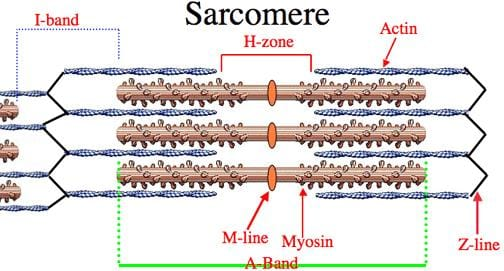QUESTION: 153

People living at sea level have around 5 million RBC per cubic millimeter of their blood whereas those living at an altitude to 5400 metres have around 8 million.This is because at high altitude:

Solution:

At high altitudes, the atmospheric O2 level is less and hence, more RBCs are needed to absorb the required amount of O2 to survive. That is why the people living at sea level have around 5 million RBC/mm3 of their blood whereas those living at an altitude of 5400 meters have around 8 million RBC/mm3 of their blood.

QUESTION: 154

Blood of AB group cannot be given to B group patient because:

Solution:

Persons with blood group B have B antigen on the surface of their RBCs and anti A antibodies (against A antigen) in their plasma. The person with AB blood group possesses both the antigens A and B on the surface of RBCs. If AB blood group is given to B group patient, agglutination occurs between anti A antibodies and A antigen.

QUESTION: 155

Which ones of the following statements are wrong?

(i)   RBC, WBC and blood platelets are produced by bone marrow.

(ii)   Neutrophils bring about destruction and detoxification of toxins of protein origin.

(iii)   Important function of lymphocytes is to produce antibodies.

Solution:

Neutrophil undergo self destruction. It is a vital immune system neutrophils are white blood cells and protein detoxification is a process in which neutrophils doesn't take part.

QUESTION: 156

Which of the following statements is false ?

Solution:
• This statement is false. Hence, ADH doesn't help in water elimination, therefore making urine Hypotonic.
• When ADH secretion is less, water absorption is less and this is the leading cause of hypotonic.
• Therefore, high ADH secretion doesn't turn urine Hypotonic but less ADH makes it hypotonic.
QUESTION: 157

The slow twitch muscle fibres which are rich in myoglobin and have abundant mitochondria are:

Solution:

Red muscle fibres:

• These muscle fibres are red as they are rich in myoglobin.
• These muscles have less sarcoplasmic reticulum.
• Mitochondria are more in number.
• They are also called as Aerobic muscles.
• These muscle fibres are thinner.
• They have rich blood supply.
• These muscle fibers contract slowly, but for longer duration.
• These are called Oxidative fibres.

Example: Extensor muscles of human back, flight muscles of kites.

QUESTION: 158

One common example of simple reflex is :

Solution:

Simple or unconditional reflexes are present in an individual right from birth. They are specific, predictable, purposeful and have survival value, e.g. breast feeding and swallowing in newly born babies and blinking of eyes are examples of unconditioned reflexes.

(a), (b) and (c) are the examples of conditional reflexes and are not present at birth but develop later in life through learning habit.

QUESTION: 159

A person is having problems with calcium and phosphorus metabolism in his body. Which one of the following glands may not be functioning properly ?

Solution:

The parathormone secreted by parathyroid hormone regulates the calcium and phosphate balance between the blood and other tissues.

QUESTION: 160

Malignant tertian malaria is caused by :

Solution:

Malaria is caused by obligate intracellular protozoan parasites of the genus "Plasmodium". The four species of human malarial parasites are Plasmodiumvivax, P falciparum, P malariae, and P ovale.

Malignant Malaria: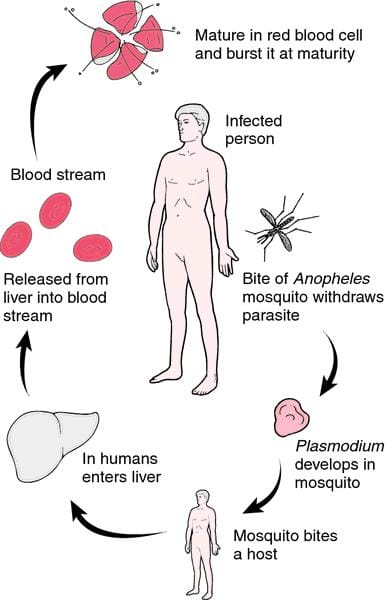QUESTION: 161

Angiotensinogen is a protein produced and secreted by :

Solution:

Angiotensinogen is a plasma protein produced and secreted by liver cells. It is a sole precursor of all angiotensin peptide. Juxtaglomerular cells secrete renin which acts on angiotensinogen to release angiotensin I and then it is converted to angiotensin II by the action of enzyme angiotensin-converting enzymes.

So, the correct answer is 'Liver cells'.

QUESTION: 162

Cocaine is derived from :

Solution:

Erythroxylum coca - also known as Erythroxylon coca has a naturally occurring alkaloid in its leaves.  That alkaloid is called "cocaine."

QUESTION: 163

Smoking addiction is harmful because it produces polycyclic aromatic hydrocarbons, which cause:

Solution:

Polycyclic aromatic hydrocarbons are carcinogenic which cause cancer.

QUESTION: 164

Match the entries in Column-I with those of Column-II and choose the correct answer.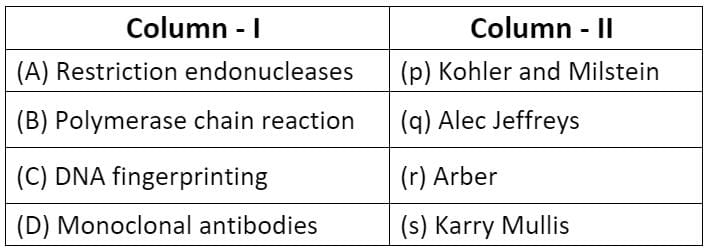Solution:

A. Restriction endonucleases - r. Arber
B. Polymerase Chain Reaction - s. Karry Mullis
C. DNA fingerprinting - q. Alec Jeffreys
D. Monoclonal antibodies - p. Kohler and Milstein

So the correct option is 'A−r,B−s,C−q,D−p'.

QUESTION: 165

Human body temperature is maintained by :

Solution:

Body temperature refers to the temperature in the hypothalamus and in the vital internal organs. Because we cannot measure the temperature inside these organs, temperature is taken on parts of the body that are more accessible. But these measurements are always slightly inaccurate.

QUESTION: 166

Which of the following is the contractile protein of a muscle ?

Solution:

Myosin is the protein that makes up the thick filaments and it comprises approximately 50-55% of the muscle protein by weight.

So, the correct answer is 'Myosin'.

QUESTION: 167

Cranium of human contains:

Solution:

Cranium of the human body consist of 8 bones. there are 1 frontal, 2 parietal, 2 temporal, 1 occipital, 1 sphenoid and 1 ethmoid bone.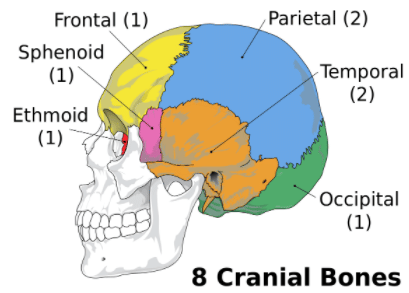QUESTION: 168

A cell-coded protein that is formed in response to infection with most animal viruses, is called :

Solution:

Interferons blocks protein synthesis and cell growth, only attach to cells infected by viruses, prevent modification of viruses inside the cells.

QUESTION: 169

The antibodies are :

Solution:

Antibodies are large Y-shaped proteins. They are recruited by the immune system to identify and neutralize foreign objects like bacteria and viruses.

QUESTION: 170

Eco RI cleaves the DNA strands to produce :

Solution:
• Sticky ends are formed when the restriction enzymes cut the DNA molecule at such a position that it leaves an overhanging stretch of the nitrogenous base which can pair with the complementary base pairs.
• EcoRI cut through the DNA strands at nucleotides that overhangs at the end. This overhanging nucleotide strand is called a sticky end because it can easily bond with complementary DNA fragments. So, the correct answer is option B.
QUESTION: 171

Short-lived immunity acquired from mother to foetus across placenta or through mother’s milk to the infant is categorized as:

Solution:

Passive immunity is the one in which the individual acquires pre-formed antibodies from outside and so is the case of the immunity that foetus/infant/acquires from mother via placenta/mother's milk.

QUESTION: 172

Which gas was probably least abundant in the early atmosphere?

Solution:
• Scientists believe that the Earth was formed about 4.5 billion years ago. Its early atmosphere was probably formed from the gases given out by volcanoes. It is believed that there was intense volcanic activity for the first billion years of the Earth's existence.
• The early atmosphere was probably mostly carbon dioxide, with little or no oxygen. There were smaller proportions of water vapour, ammonia and methane. As the Earth cooled down, most of the water vapour condensed and formed the oceans.
QUESTION: 173

‘YAC’ refers to:

Solution:

Yeast artificial chromosomes are genetically engineered chromosomes derived from the DNA of the yeast, Saccharomyces cerevisiae, which is then ligated into a bacterial plasmid.

QUESTION: 174

Damage to thymus in a child may lead to:

Solution:
• Thymus gland is found in the upper part of thorax near the heart. It is a bilobed pinkish gland. At the time of birth, it is a prominent gland but in the adult it gradually atrophics.
• This gland secrets thymosin hormone, thymic humoral factor, thymic factor and thymopoietin. Proliferation of lymphocytes and differentiation of these lymphocytes into a variety of clones are induced by these factors. These clones are differentially specialized to destroy and pathogens.
• Thus thymus gland brings fourth T -lymphocytes for cell mediated immunity.
QUESTION: 175

The peritubular capillaries of the nephron arise from the:

Solution:

The efferent arterioles are the arterioles that take blood away from the glomerulus. The efferent arterioles give rise to peritubular capillaries. peritubular capillaries are tiny blood vessels, that travel alongside nephrons allowing reabsorption and secretion between blood and the inner lumen of the nephron.

Nephron: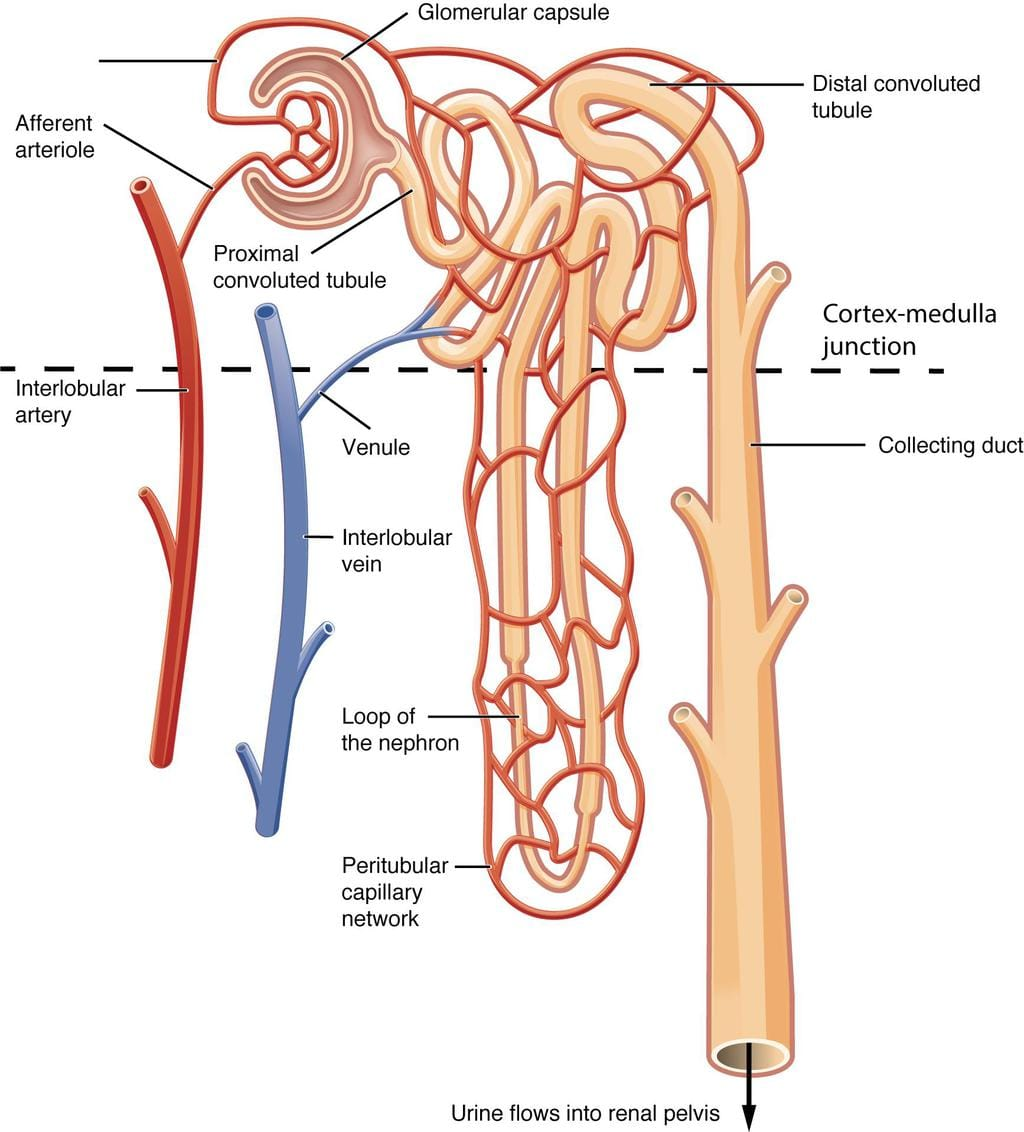QUESTION: 176

Oral contraceptive prevents pregnancy by:

Solution:

Oral contraceptives (birth-control pills) are used to prevent pregnancy. Estrogen and progestin are two female sex hormones. Combinations of estrogen and progestin work by preventing ovulation (the release of eggs from the ovaries).

QUESTION: 177

A person may die due to allergic reaction or an anaphylactic shock which is characterized by:

Solution:
• Anaphylaxis is a severe allergic reaction that occurs rapidly and causes a life-threatening response involving the whole body. This reaction can lead to difficulty breathing and shock ultimately leading to death.
• Most frequently, severe allergic reactions occur due to reactions to food, insect stings, or medications (immunologic causes), but they can also occur due to non-immunologic causes.
QUESTION: 178

During muscle contraction in humans, the______.

Solution:
• During muscular contraction, the myosin heads pull the actin filaments toward one another resulting in a shortened sarcomere. While the I band and H zone will disappear or shorten, the A band length will remain unchanged. This is because the A band corresponds to the full length of the myosin filament, or thick filament. Since the myosin filament does not actually change length, the A band remains constant.
• The I band corresponds to the region of action that does not overlap with myosin. The length of the actin filament does not change during contraction, but the region of overlap increases. This results in a decrease of the non-overlapped I band.
• The H zone refers to the region of myosin that is not overlapped by action. As the region of overlap grows, the H zone shrinks.
QUESTION: 179

One of the most frequently used techniques in DNA fingerprinting is:

Solution:

Variable Number of Tandem Repeat (VNTR) loci are chromosomal regions in which a short DNA sequence motif (such as GC or AGCT) is repeated a variable number of times end-to-end at a single location (tandem repeat).

QUESTION: 180

Select the correct option with respect to cockroaches:

Solution:

Malpighian tubules are the main excretory structures in cockroach. They extract nitrogenous wastes and water from haemolymph and reabsorb certain salts resulting in precipitation of uric acid. So, cockroach is uricotelic. Males have paired anal styles on 9th abdominal stemite which are absent in females.Use Code STAYHOME200 and get INR 200 additional OFF Use Coupon Code